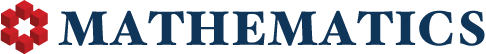## Up vs Down votes by day of week of Question or Answer

10

(click on this box to dismiss)Q&A for people studying math at any level and professionals in related fields

``````-- Up vs Down votes by day of week of Question or Answer

SELECT
CASE WHEN PostTypeId = 1 THEN 'Question' ELSE 'Answer' END As [Post Type],
DATENAME(WEEKDAY, p.CreationDate) AS Day,
Count(*) AS Amount,
SUM(CASE WHEN VoteTypeId = 2 THEN 1 ELSE 0 END) AS UpVotes,
SUM(CASE WHEN VoteTypeId = 3 THEN 1 ELSE 0 END) AS DownVotes,
CASE WHEN SUM(CASE WHEN VoteTypeId = 3 THEN 1 ELSE 0 END) = 0 THEN NULL
ELSE (CAST(SUM(CASE WHEN VoteTypeId = 2 THEN 1 ELSE 0 END) AS float) / CAST(SUM(CASE WHEN VoteTypeId = 3 THEN 1 ELSE 0 END) AS float))
END AS UpVoteDownVoteRatio
FROM
Votes v JOIN Posts p ON v.PostId=p.Id
WHERE
PostTypeId In (1,2)
AND
VoteTypeId In (2,3)
GROUP BY
PostTypeId, DATEPART(WEEKDAY, p.CreationDate), DATENAME(WEEKDAY, p.CreationDate)
ORDER BY
PostTypeId, DATEPART(WEEKDAY, p.CreationDate)``````

### Enter Parameters

Options:
-Hold tight while we fetch your results
:records returned in :time ms:cached